Next: Multiplexers and Decoders Up: Combinational Logic Previous: Signal Race

From basic gates, we will develop a full adder circuit that adds two binary numbers. Consider adding two 2-bit binary numbers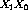and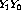.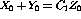, where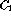is the carry bit. The truth table for all combinations of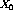and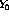is shown in table 7.5.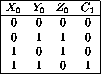Table 7.5:  The binary addition of two 2-bit numbers. The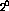column.

From the truth table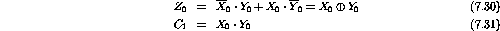The mechanization of these two equation is shown in figure 7.7.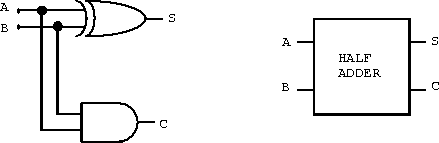Figure 7.7:  A mechanization of the half adder using an EOR and an AND gate.

This circuit is known as the half adder. It can not handle the addition of any two arbitrary numbers because it does not allow the input of a carry bit from the addition of two previous digits. A circuit that can handle these three inputs can perform the addition of any two binary numbers.

The truth table for three input variables is shown in figure 7.8.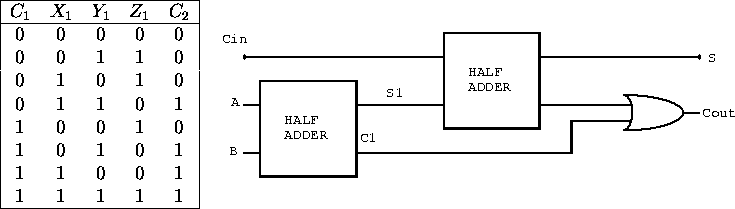Figure 7.8:  The binary addition of two 2-bit numbers. The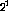column.

From the truth table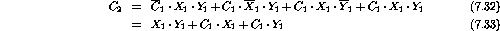This is known as majority logic. And a majority detector is shown in figure 7.9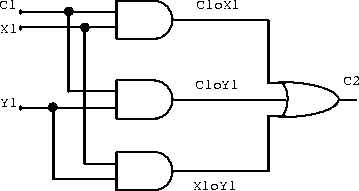Figure 7.9:  A mechanization of the majority detector.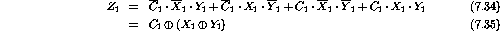The following device (figure 7.10) is known as a full adder and is able to add three single bits of information and return the sum bit and a carry-out bit.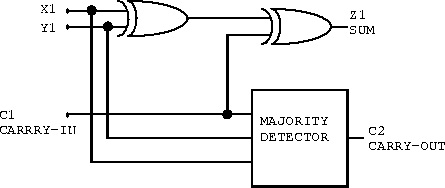Figure 7.10:  The full adder mechanization.

The circuit shown in figure 7.11 is able to add any two numbers of any size. The inputs are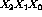and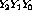, and the output is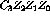.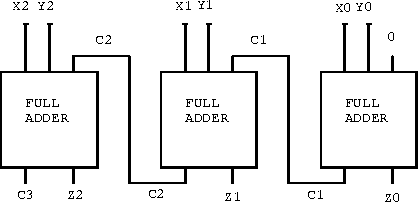Figure 7.11:  A circuit capable of adding two 3-bit numbers.

Example: If the input to the circuit in figure 7.12 is written as a number ABCD, write the nine numbers that will yield a true Q.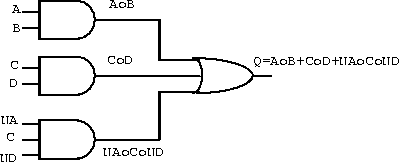Figure 7.12:  A typical logic function.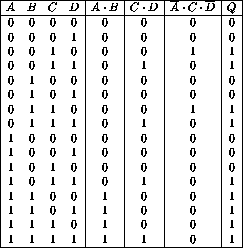Table 7.6: The truth table for the typical logic function example.

ABCD=(2,3,6,7,11,12,13,14,15) gives Q true.

Example: Using the 2's complement convention, the 3-bit number ABC can represent the numbers from -3 to 3 as shown in table 7.7 (ignore -4). Assuming that A, B, C and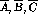are available as inputs, the goal is to devise a circuit that will yield a 2-bit output EF that is the absolute value of the ABC number. You have available only two- and three-input AND and OR gates.

1. Fill a truth table with the ABC and EF bits.

The truth table is shown in table 7.7.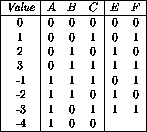Table 7.7:  Truth table with for the ABC and EF bits.

2. Write a Boolean algebra expression for E and for F.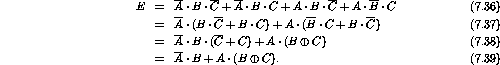3. Mechanize these expressions.

The mechanized expressions are shown in figure 7.13.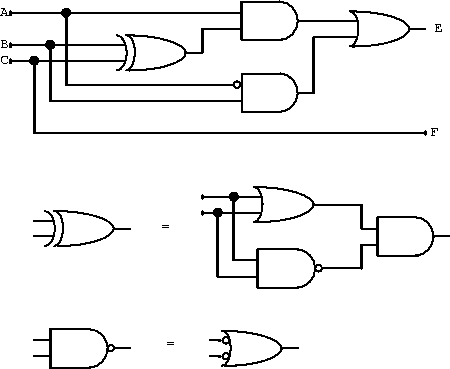Figure 7.13:  Mechanization for the ABC and EF bits.

Example: Suppose that the 2-bit binary number AB must be transmitted between devices in a noisy environment. To reduce undetected errors introduced by the transmission, an extra bit P is often included to add redundancy to the information. Assume that P is set true or false as needed to make an odd number of true bits in the resulting 3-bit number ABP. When the number is received, logic circuits are required to generate an error signal E whenever the odd number of bits condition is not met.

1. Develop a truth table of E in terms of A, B and P.

The truth table is shown in table 7.8.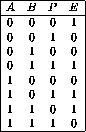Table 7.8:  Truth table for E in terms of A, B and P.

2. Write a Boolean expression for E as determined directly from the truth table.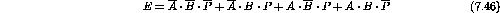3. Using De Morgan's theorem twice, reduce this expression to one EOR and one NEOR operation. (This is very similar to the half-adder problem.)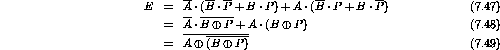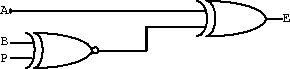Figure 7.14: Mechanization for E.Next: Multiplexers and Decoders Up: Combinational Logic Previous: Signal Race

Doug Gingrich
Tue Jul 13 16:55:15 EDT 1999